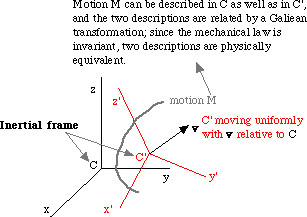Spacetime

Galilean Principle of Relativity and Galilean Transformation

Galilean Principle of Relativity and Galilean Transformation

The following is indeed an elementary point, but since some students have felt puzzled with the expression "Galilean invariance", I should like to add this note.

Inertial frames exist innumerably, and non-inertial frames also exist innumerably. In any inertial frame, mechanical laws (such as the Newtonian first or second law of motion) do not change. Thus there is no priviledged inertial frame with respect to mechanical laws; or any inertial frames are (physicallly) equivalent with respect to mechanical laws. This is the Galilean Principle of Relativity.

Notice that although Newton's equation of motion does not change, Maxwell's equations of electromagnetism do change, depending on which inertial frame one is adopting. Now, take an inertial frame C (coordinate system) and describe a motion. If we take another inertial frame C' (moving with a constant velocity relative to the former), the same motion is described differently in the new coordinate system. But the two descriptions are related with each other by such relations as

x' = x - vt (v is the x-component of the constant velocity and t is the time),

and we call any transformation of this nature, Galilean Transformation. And sometimes we say that the Newtonian laws are invariant with respect to the Galilean Transformation. Notice that any acceleration in C remains the same (invariant) even if it is redescribed in C'.